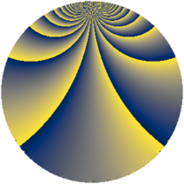# Properties

 Label 1849.4.iLevel $1849$ Weight $4$ Character orbit 1849.i Rep. character $\chi_{1849}(44,\cdot)$ Character field $\Q(\zeta_{43})$ Dimension $19824$ Sturm bound $630$

# Related objects

## Defining parameters

 Level: $$N$$ $$=$$ $$1849 = 43^{2}$$ Weight: $$k$$ $$=$$ $$4$$ Character orbit: $$[\chi]$$ $$=$$ 1849.i (of order $$43$$ and degree $$42$$) Character conductor: $$\operatorname{cond}(\chi)$$ $$=$$ $$1849$$ Character field: $$\Q(\zeta_{43})$$ Sturm bound: $$630$$

## Dimensions

The following table gives the dimensions of various subspaces of $$M_{4}(1849, [\chi])$$.

Total New Old
Modular forms 19908 19908 0
Cusp forms 19824 19824 0
Eisenstein series 84 84 0

## Trace form

 $$19824q - 45q^{2} - 39q^{3} - 1915q^{4} - 59q^{5} - 13q^{6} - 31q^{7} - 31q^{8} - 4273q^{9} + O(q^{10})$$ $$19824q - 45q^{2} - 39q^{3} - 1915q^{4} - 59q^{5} - 13q^{6} - 31q^{7} - 31q^{8} - 4273q^{9} - 97q^{10} + 57q^{11} + 53q^{12} - 93q^{13} - 1476q^{14} - 11q^{15} - 7583q^{16} + 137q^{17} - 261q^{18} + 3158q^{19} - 259q^{20} + 65q^{21} + 259q^{22} - 3623q^{23} + 315q^{24} - 11597q^{25} - 37q^{26} - 63q^{27} + 441q^{28} - 459q^{29} - 5666q^{30} + 2119q^{31} - 259q^{32} - 551q^{33} + 11q^{34} + 5465q^{35} - 16369q^{36} + 137q^{37} - 305q^{38} - 431q^{39} - 789q^{40} - 103q^{41} - 11q^{42} + 43q^{43} + 1309q^{44} - 39q^{45} - 1037q^{46} - 353q^{47} + 709q^{48} - 23267q^{49} - 1977q^{50} - 451q^{51} - 195q^{52} - 335q^{53} + 619q^{54} + 329q^{55} + 1051q^{56} - 436q^{57} + 1783q^{58} - 1235q^{59} + 3335q^{60} + 913q^{61} - 1549q^{62} + 333q^{63} - 30511q^{64} + 369q^{65} + 53364q^{66} + 321q^{67} - 6312q^{68} + 2325q^{69} + 2577q^{70} - 367q^{71} + 40641q^{72} - 1535q^{73} - 1743q^{74} + 2346q^{75} - 5471q^{76} - 659q^{77} - 2218q^{78} + 215q^{79} - 2411q^{80} - 39419q^{81} + 42367q^{82} + 1413q^{83} - 3709q^{84} + 25q^{85} + 387q^{86} + 14691q^{87} - 1251q^{88} - 2683q^{89} + 52475q^{90} + 3005q^{91} - 1433q^{92} - 1887q^{93} + 22213q^{94} - 5898q^{95} + 1077q^{96} - 30681q^{97} - 4093q^{98} - 12973q^{99} + O(q^{100})$$

## Decomposition of $$S_{4}^{\mathrm{new}}(1849, [\chi])$$ into newform subspaces

The newforms in this space have not yet been added to the LMFDB.